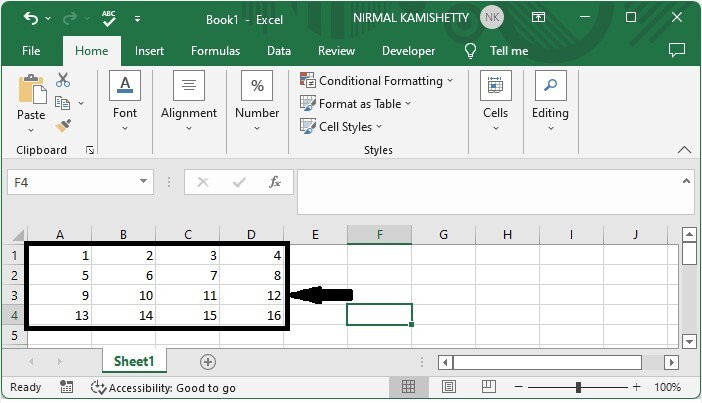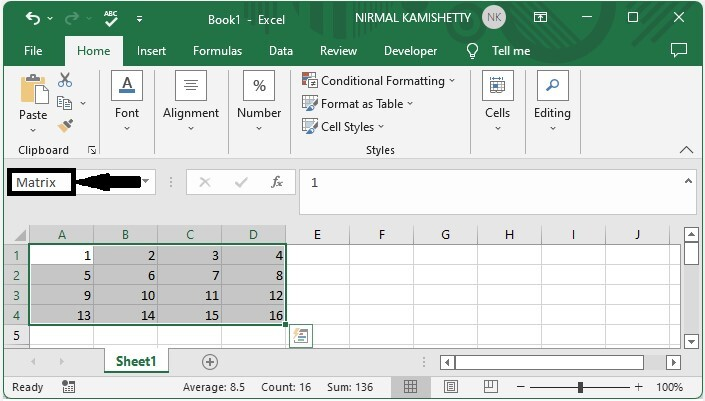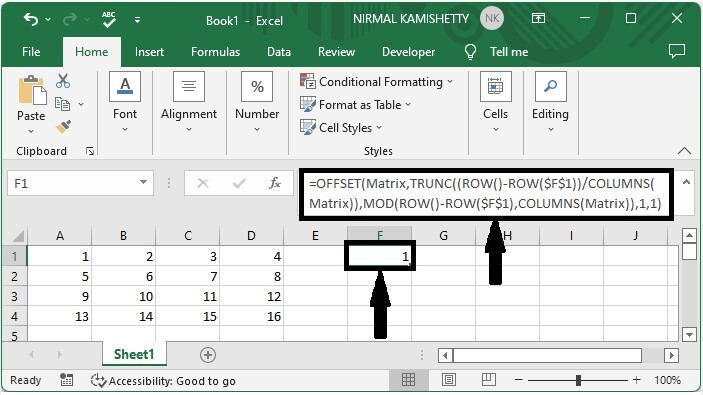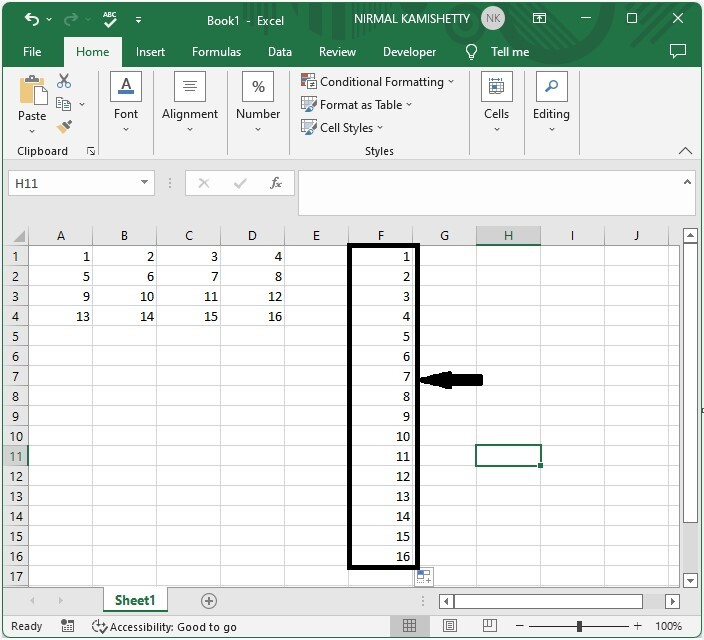# How to Convert Matrix to Vector or Single Column in Excel?

A matrix is a rectangular array with n rows and m columns. Sometimes in Excel, when we have matrices of data, we want to convert them into a single column or single row. We can solve this using the formulas supported by Excel. Read this tutorial to learn how you can convert a matrix to a vector or single column in Excel. If you try to do it manually, then it can be a time‑consuming process as we need to copy each row manually and paste them. So let's see a faster process. The only condition for the matrix is that all the values must be filled in as whole numbers.

## Converting a Matrix to a Single Column in Excel

Here we will name the data first, then use the OFFSET formula to paste one value, then drag down to fill all the values. Let's look at a simple procedure for converting a matrix to a single column in Excel.

### Step 1

Consider an Excel sheet with a matrix similar to the one shown below.First, select all the values and name them Matrix, as shown in the below image.### Step 2

Now click on the empty cell from where you want to start your list, enter the formula as =OFFSET(Matrix,TRUNC((ROW()-ROW($F$1))/COLUMNS(Matrix)),MOD(ROW()-ROW($F$1),COLUMNS(Matrix)),1,1), and click Enter to get our value as shown in the below image. In the formula, F1 is the cell into which we are entering the formula, and matrix is the name of our values.### Step 3

Drag down from the first value using the auto-fill handle until zeros appear and our final the result will be similar to the below image.This is how we can convert a matrix to a single-column list in Excel using the formulas.

If we need to display the list based on column. We can use the formula as

=OFFSET(Matrix,MOD(ROW()-ROW($G$1),ROWS(Matrix)),TRUNC((ROW()-ROW($G$1))/ROWS(Matrix)),1,1)

If we want to convert the matrix to a single row, we can use the same method, but we must change the formula.

=OFFSET(Matrix,TRUNC((COLUMN()-COLUMN($A$7))/COLUMNS(Matrix)),MOD((COLUMN()-COLUMN($A$7)),COLUMNS(Matrix)),1,1)

## Conclusion

In this tutorial, we used a simple example to demonstrate how you can convert a matrix to a vector or single column in Excel.

Updated on: 06-Mar-2023

2K+ Views